# How to use Goal Seek in Excel for What-If analysis

The tutorial explains how to use Goal Seek in Excel 2016, 2013 and 2010 to get the formula result you want by changing an input value.

What-If Analysis is one of the most powerful Excel features and one of the least understood. In most general terms, What-If Analysis allows you to test out various scenarios and determine a range of possible outcomes. In other words, it enables you to see the impact of making a certain change without changing the real data. In this particular tutorial, we will focus on one of Excel's What-If Analysis tools - Goal Seek.

## What is Goal Seek in Excel?

Goal Seek is Excel's built-in What-If Analysis tool that shows how one value in a formula impacts another. More precisely, it determines what value you should enter in an input cell to get the desired result in a formula cell.

The best thing about Excel Goal Seek is that it performs all calculations behind the scenes, and you are only asked to specify these three parameters:

• Formula cell
• Target/desired value
• The cell to change in order to achieve the target

The Goal Seek tool is especially useful for doing sensitivity analysis in financial modeling and is widely used by management majors and business owner. But there are many other uses that may prove helpful to you.

For instance, Goal Seek can tell you how much sales you have to make in a certain period to reach \$100,000 annual net profit (example 1). Or, what score you must achieve for your last exam to receive an overall passing score of 70% (example 2). Or, how many votes you need to get in order to win the election (example 3).

On the whole, whenever you want a formula to return a specific result but are not sure what input value within the formula to adjust to get that result, stop guessing and use the Excel Goal Seek function!

Note. Goal Seek can process only one input value at a time. If you are working on an advanced business model with multiple input values, use the Solver add-in to find the optimal solution.

## How to use Goal Seek in Excel

The purpose of this section is to walk you through how to use the Goal Seek function. So, we'll be working with a very simple data set: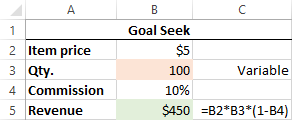The above table indicates that if you sell 100 items at \$5 each, minus the 10% commission, you will make \$450. The question is: How many items do you have to sell to make \$1,000?

Let's see how to find the answer with Goal Seek:

1. Set up your data so that you have a formula cell and a changing cell dependent on the formula cell.
2. Go to the Data tab > Forecast group, click the What if Analysis button, and select Goal Seek…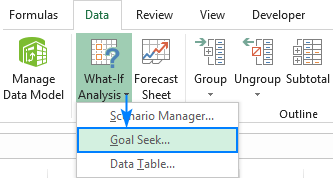3. In the Goal Seek dialog box, define the cells/values to test and click OK:
• Set cell - the reference to the cell containing the formula (B5).
• To value - the formula result you are trying to achieve (1000).
• By changing cell - the reference for the input cell that you want to adjust (B3).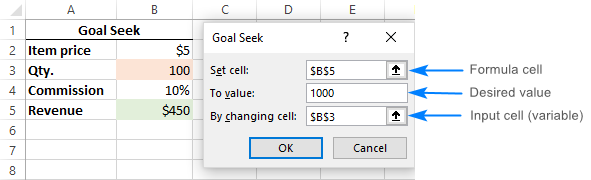4. The Goal Seek Status dialog box will appear and let you know if a solution has been found. If it succeeded, the value in the "changing cell" will be replaced with a new one. Click OK to keep the new value or Cancel to restore the original one.

In this example, Goal Seek has found that 223 items (rounded up to the next integer) need to be sold to achieve a revenue of \$1,000.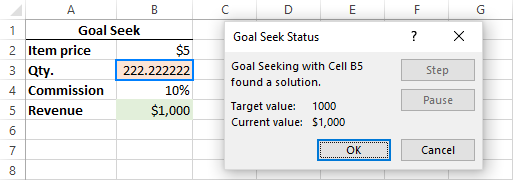If you are not sure you will be able to sell that many items, then maybe you can reach the target revenue by changing the item price? To test this scenario, do Goal Seek analysis exactly as described above except that you specify a different Changing cell (B2):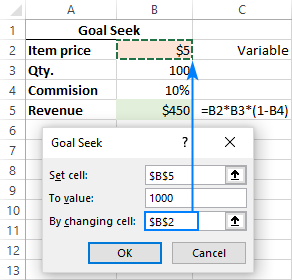As the result, you will find out that if you increase the unit price to \$11, you can reach \$1,000 revenue by selling only 100 items: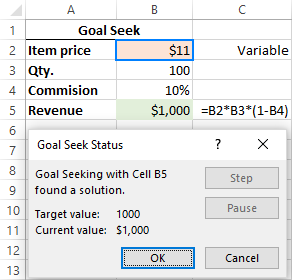Tips and notes:

• Excel Goal Seek does not change the formula, it only changes the input value that you supply to the By changing cell box.
• If Goal Seek is not able to find the solution, it displays the closest value it has come up with.
• You can restore the original input value by clicking the Undo button or pressing the Undo shortcut (Ctrl + Z).

## Examples of using Goal Seek in Excel

Below you will find a few more examples of using the Goal Seek function in Excel. The complexity of your business model does not really matter as long as your formula in the Set cell depends on the value in the Changing cell, directly or through intermediate formulas in other cells.

### Example 1: Reach the profit goal

Problem: It is a typical business situation - you have the sales figures for the first 3 quarters and you want to know how much sales you have to make in the last quarter to achieve the target net profit for the year, say, \$100,000.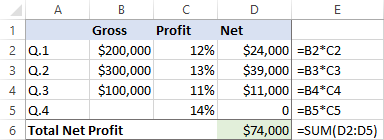Solution: With the source data organized like shown in the screenshot above, set up the following parameters for the Goal Seek function:

• Set cell - the formula that calculates the total net profit (D6).
• To value - the formula result you are looking for (\$100,000).
• By changing cell - the cell to contain the gross revenue for quarter 4 (B5).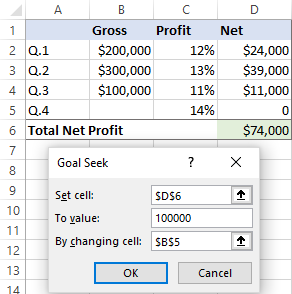Result: The Goal Seek analysis shows that in order to obtain \$100,000 annual net profit, your fourth-quarter revenue must be \$185,714.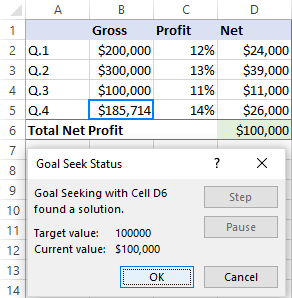### Example 2: Determine the exam passing score

Problem: At the end of the course, a student takes 3 exams. The passing score is 70%. All the exams have the same weight, so the overall score is calculated by averaging the 3 scores. The student has already taken 2 out of 3 exams. The question is: What score does the student need to get for the third exam to pass the entire course?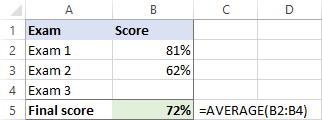Solution: Let's do Goal Seek to determine the minimum score on exam 3:

• Set cell - the formula that averages the scores of the 3 exams (B5).
• To value - the passing score (70%).
• By changing cell - the 3rd exam score (B4).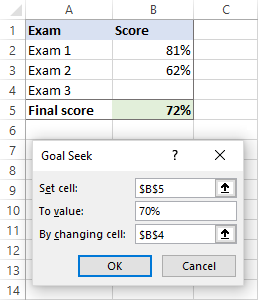Result: In order get the desired overall score, the student must achieve a minimum of 67% on the last exam: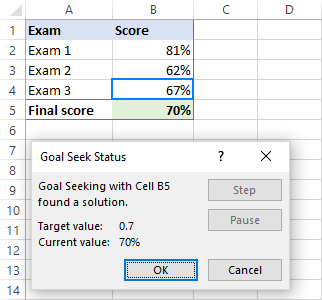### Example 3: What-If analysis of the election

Problem: You are running for some elected position where a two-thirds majority (66.67% of votes) is required to win the election. Assuming there are 200 total voting members, how many votes do you have to secure?

Currently, you have 98 votes, which is quite good but not sufficient because it only makes 49% of the total voters: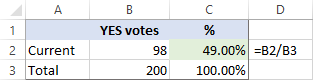Solution: Use Goal Seek to find out the minimum number of "Yes" votes you need to get:

• Set cell - the formula that calculates the percentage of the current "Yes" votes (C2).
• To value - the required percentage of "Yes" votes (66.67%).
• By changing cell - the number of "Yes" votes (B2).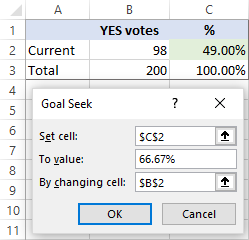Result: What-If analysis with Goal Seek shows that to achieve the two-thirds mark or 66.67%, you need 133 "Yes" votes:## Excel Goal Seek not working

Sometimes Goal Seek is not able to find a solution simply because it does not exist. In such situations, Excel will get the closest value and inform you that Goal Seeking may not have found a solution: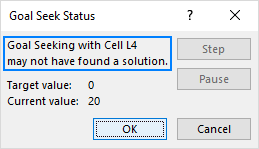If you are certain that a solution to the formula you are trying to resolve does exist, check out the following troubleshooting tips.

### 1. Double check Goal Seek parameters

First off, make sure the Set cell refers to the cell containing a formula, and then, check if the formula cell depends, directly or indirectly, on the changing cell.

In your Excel, click File > Options > Formulas and change these options:

• Maximum Iterations - increase this number if you want Excel to test more possible solutions.
• Maximum Change - decrease this number if your formula requires more accuracy. For example, if you are testing a formula with an input cell equal to 0 but Goal Seek stops at 0.001, setting Maximum Change to 0.0001 should fix the issue.

The below screenshot shows the default iteration settings: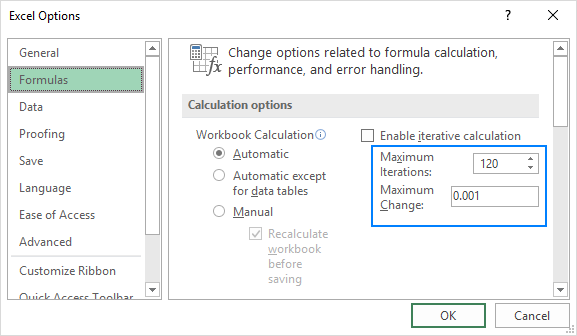### 3. No circular references

For Goal Seek (or any Excel formula) to work properly, the involved formulas should not be co-dependent on each other, i.e. there should be no circular references.

That's how you perform What-If analysis in Excel with the Goal Seek tool. I thank you for reading and hope to see you on our blog next week!

### 12 responses to "How to use Goal Seek in Excel for What-If analysis"

1.John says:

This is great to read at work for application, thanks!

2.Edickoff says:

I join. So happens. Let's discuss this question.

3.sanoj says:

Can we refer to a cell for the To value?

•Svetlana Cheusheva (Ablebits.com Team) says:

Hi Sanoj,

Nope. The To field needs to be a value (integer or decimal number), not a cell reference.

4.Michael says:

Is it possible to What if / Goal seek across multiple cells - like a copy / paste approach ?

5.Anwar says:

While using Goal Seak function, It deletes the formula in the cell and the cell which is required to be changes is not changed.
For Example Cell A contain a vaule say 10
Cell B conatin a value say 2
Cell C has a formula A X B, the result is 20
Now When I apply goal seak formula in Cell to Find what will will be the value in cell A, If I need a result of 200 in Cell C. It deltets the the formula in cell C and does not chnge the value in cell A also

•Ian Whitiker Gomo says:

i AM FACING THE SAME PROBLEM

6.Mat says:

Excel 3013?

•Svetlana Cheusheva (Ablebits.com Team) says:

Just a misprint :) Fixed, thank you!

7.Josh says:

How to do with goal seek error “ cell need a format.

8.James Mattson says:

Would this work for the following problems:
You have a list of invoices (and amounts) and two credit card charges but no documentation which charges paid which bills... Can goal seek work backwards from the charge to determine which invoices were included in each payment?

9.PA says:

Very nice